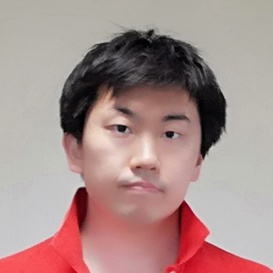AOJ

# # Problem

http://judge.u-aizu.ac.jp/onlinejudge/description.jsp?id=DPL_3_A

# # Time complexity

$O(N^2)$

# # Solution

#define MAX_N 1401

Int H, W;
bool S[MAX_N][MAX_N];
Int A[MAX_N][MAX_N];

void input() {
cin >> H >> W;
loop(h,0,H) {
loop(w,0,W) {
cin >> S[h][w];
A[h][w] = -1;
}
}
}

void solve() {
Int max_ = 0;
loop(h,0,H) {
A[h] = (S[h]) ? 0 : 1;
max_ |= A[h];
}
loop(w,0,W) {
A[w] = (S[w]) ? 0 : 1;
max_ |= A[w];
}

loop(h,1,H) {
loop(w,1,W) {
if (S[h][w]) {
A[h][w] = 0;
continue;
}

A[h][w] = min(A[h-1][w], min(A[h][w-1], A[h-1][w-1])) + 1;
if (A[h][w] > max_) max_ = A[h][w];
}
}

cout << max_*max_ << endl;
}

int main(void) {
input();
solve();
}Remote freelancer. A web and mobile application enginner.
Traveling around the world based on East Asia.
I'm looking forward to your job offers from all over the world!

Offer jobs or contact me!